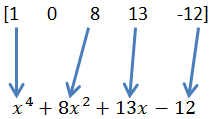Home MathLab Matlab Polynomial: Division and Multiplication

# Matlab Polynomial: Division and Multiplication

We have recently learned how to solve polynomial equations in Matlab, now we are going to visit a trick that will help you learn how to do Division and Multiplication of polynomial using Matlab.

## Polynomial Multiplication example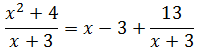## Matlab Polynomial

Multiplication of polynomial can be a very dreary task, so do the division of polynomial. Matlab uses the functions conv and deconv to help you do these tasks with the least commotion possible, and most importantly with the assurance to find the right result the quickest way possible.

Let get on some examples, those will help easily learn how to make use of conv (for multiplication) and deconv (for division).

Caution! We will not show you how to manually solve polynomial multiplication and polynomial division manually here. Matlab will help you do it, but it will not show you how to do it, so at this point we supposed you know how it is done. Check how to do it manually if you do not.

### Example 1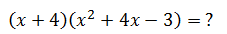The code

```s=[1 4];
t=[1 4 -3];
conv(s,t)```

Which returns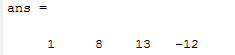Rewritten, it gives the following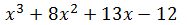### Example 2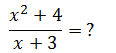The code

```c=[1 0 4];
u=[1 3];
[p,r]=deconv(c,u)% r is the remainder of the division```

Which returns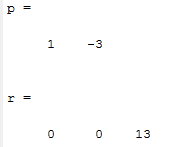Rewritten, it gives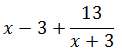### A bit of clarity

Top-down conversion. Here we simply need to indicate the coefficient and their position in the polynomial equation.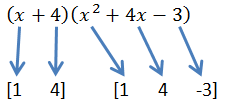Bottom-up conversion. Here we need to read Matlab outputs and rewrite it the way it is supposed to be, and it goes like this.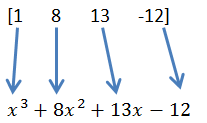Remember you need to put a zero, in case an order is not represented in the equation. See the example below